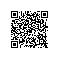# MCMC(三)MCMC采样和M-H采样

在MCMC(二)马尔科夫链中我们讲到给定一个概率平稳分布ππ, 很难直接找到对应的马尔科夫链状态转移矩阵PP。而只要解决这个问题，我们就可以找到一种通用的概率分布采样方法，进而用于蒙特卡罗模拟。本篇我们就讨论解决这个问题的办法：MCMC采样和它的易用版M-H采样。
1. 马尔科夫链的细致平稳条件

$$\pi(i)P(i,j) = \pi(j)P(j,i)$$

$$\sum\limits_{i=1}^{\infty}\pi(i)P(i,j) = \sum\limits_{i=1}^{\infty} \pi(j)P(j,i) = \pi(j)\sum\limits_{i=1}^{\infty} P(j,i) = \pi(j)$$

$$\pi P = \pi$$

$$\pi(i)Q(i,j) \neq \pi(j)Q(j,i)$$

2. MCMC采样

$$\pi(i)Q(i,j) \neq \pi(j)Q(j,i)$$

$$\pi(i)Q(i,j)\alpha(i,j) = \pi(j)Q(j,i)\alpha(j,i)$$

]

$$\alpha(i,j) = \pi(j)Q(j,i)$$

$$\alpha(j,i) = \pi(i)Q(i,j)$$

$$P(i,j) = Q(i,j)\alpha(i,j)$$

1. 输入我们任意选定的马尔科夫链状态转移矩阵$Q$，平稳分布$π(x)$，设定状态转移次数阈值$n_1$，需要的样本个数$n_2$
2. 从任意简单概率分布采样得到初始状态值$x_0$
3. for $t=0$ to $n_1 +n_2-1$:

1. 从条件概率分布$Q(x|x_t)$中采样得到样本$x∗$
2. 从均匀分布采样$u \sim uniform[0,1]$
3. 如果$u < \alpha(x_t,x_{*}) = \pi(x_{*})Q(x_{*},x_t)$, 则接受转移$x_t \to x_{*}$,即$x_{t+1}= x_{*}$
4. 否则不接受转移，$t=max(t-1,0)$

3. M-H采样
M-H采样是Metropolis-Hastings采样的简称，这个算法首先由Metropolis提出，被Hastings改进，因此被称之为Metropolis-Hastings采样或M-H采样
M-H采样解决了我们上一节MCMC采样接受率过低的问题。

$$\pi(i)Q(i,j)\alpha(i,j) = \pi(j)Q(j,i)\alpha(j,i)$$

$$\pi(i)Q(i,j)\times 0.1 = \pi(j)Q(j,i)\times 0.2$$

$$\pi(i)Q(i,j)\times 0.5 = \pi(j)Q(j,i)\times 1$$

$$\alpha(i,j) = min\{ \frac{\pi(j)Q(j,i)}{\pi(i)Q(i,j)},1\}$$

1. 输入我们任意选定的马尔科夫链状态转移矩阵$Q$，平稳分布$π(x)$，设定状态转移次数阈值$n_1$，需要的样本个数$n_2$
2. 从任意简单概率分布采样得到初始状态值$x_0$
3. for $t = 0$ to $n_1 +n_2-1$:

1. 从条件概率分布$Q(x|x_t)$中采样得到样本$x_∗$
b.从均匀分布采样$u \sim uniform[0,1]$
2. 如果$u < \alpha(x_t,x_{*}) = min\{ \frac{\pi(j)Q(j,i)}{\pi(i)Q(i,j)},1\}$,则接受转移$x_t \to x_{*}$,即$x_{t+1}= x_{*}$
d.否则不接受转移，$t=max(t-1,0)$

$$\alpha(i,j) = min\{ \frac{\pi(j)}{\pi(i)},1\}$$

5. M-H采样总结
M-H采样完整解决了使用蒙特卡罗方法需要的任意概率分布样本集的问题，因此在实际生产环境得到了广泛的应用。

1. 我们的数据特征非常的多，M-H采样由于接受率计算式$\frac{\pi(j)Q(j,i)}{\pi(i)Q(i,j)}$的存在，在高维时需要的计算时间非常的可观，算法效率很低。同时$α(i,j)$一般小于1，有时候辛苦计算出来却被拒绝了。能不能做到不拒绝转移呢？
2. 由于特征维度大，很多时候我们甚至很难求出目标的各特征维度联合分布，但是可以方便求出各个特征之间的条件概率分布。这时候我们能不能只有各维度之间条件概率分布的情况下方便的采样呢？

Gibbs采样解决了上面两个问题，因此在大数据时代，MCMC采样基本是Gibbs采样的天下，下一篇我们就来讨论Gibbs采样。使用钉钉扫一扫加入圈子
+ 订阅